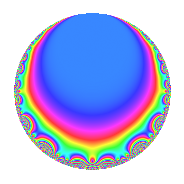# Properties

 Label 403.2.f.aLevel 403 Weight 2 Character orbit 403.f Analytic conductor 3.218 Analytic rank 1 Dimension 2 CM No Inner twists 2

# Related objects

## Newspace parameters

 Level: $$N$$ = $$403 = 13 \cdot 31$$ Weight: $$k$$ = $$2$$ Character orbit: $$[\chi]$$ = 403.f (of order $$3$$ and degree $$2$$)

## Newform invariants

 Self dual: No Analytic conductor: $$3.21797120146$$ Analytic rank: $$1$$ Dimension: $$2$$ Coefficient field: $$\Q(\sqrt{-3})$$ Coefficient ring: $$\Z[a_1, a_2]$$ Coefficient ring index: $$1$$ Sato-Tate group: $\mathrm{SU}(2)[C_{3}]$

## $q$-expansion

Coefficients of the $$q$$-expansion are expressed in terms of a primitive root of unity $$\zeta_{6}$$. We also show the integral $$q$$-expansion of the trace form.

 $$f(q)$$ $$=$$ $$q + ( -1 + \zeta_{6} ) q^{2} + \zeta_{6} q^{4} -3 q^{5} -2 \zeta_{6} q^{7} -3 q^{8} + 3 \zeta_{6} q^{9} +O(q^{10})$$ $$q + ( -1 + \zeta_{6} ) q^{2} + \zeta_{6} q^{4} -3 q^{5} -2 \zeta_{6} q^{7} -3 q^{8} + 3 \zeta_{6} q^{9} + ( 3 - 3 \zeta_{6} ) q^{10} + ( -1 - 3 \zeta_{6} ) q^{13} + 2 q^{14} + ( 1 - \zeta_{6} ) q^{16} -5 \zeta_{6} q^{17} -3 q^{18} -4 \zeta_{6} q^{19} -3 \zeta_{6} q^{20} + ( -4 + 4 \zeta_{6} ) q^{23} + 4 q^{25} + ( 4 - \zeta_{6} ) q^{26} + ( 2 - 2 \zeta_{6} ) q^{28} + ( -3 + 3 \zeta_{6} ) q^{29} - q^{31} -5 \zeta_{6} q^{32} + 5 q^{34} + 6 \zeta_{6} q^{35} + ( -3 + 3 \zeta_{6} ) q^{36} + ( 5 - 5 \zeta_{6} ) q^{37} + 4 q^{38} + 9 q^{40} + ( -7 + 7 \zeta_{6} ) q^{41} + 2 \zeta_{6} q^{43} -9 \zeta_{6} q^{45} -4 \zeta_{6} q^{46} -6 q^{47} + ( 3 - 3 \zeta_{6} ) q^{49} + ( -4 + 4 \zeta_{6} ) q^{50} + ( 3 - 4 \zeta_{6} ) q^{52} -9 q^{53} + 6 \zeta_{6} q^{56} -3 \zeta_{6} q^{58} + 6 \zeta_{6} q^{59} + \zeta_{6} q^{61} + ( 1 - \zeta_{6} ) q^{62} + ( 6 - 6 \zeta_{6} ) q^{63} + 7 q^{64} + ( 3 + 9 \zeta_{6} ) q^{65} + ( -10 + 10 \zeta_{6} ) q^{67} + ( 5 - 5 \zeta_{6} ) q^{68} -6 q^{70} + 4 \zeta_{6} q^{71} -9 \zeta_{6} q^{72} -3 q^{73} + 5 \zeta_{6} q^{74} + ( 4 - 4 \zeta_{6} ) q^{76} + 2 q^{79} + ( -3 + 3 \zeta_{6} ) q^{80} + ( -9 + 9 \zeta_{6} ) q^{81} -7 \zeta_{6} q^{82} -2 q^{83} + 15 \zeta_{6} q^{85} -2 q^{86} + ( 6 - 6 \zeta_{6} ) q^{89} + 9 q^{90} + ( -6 + 8 \zeta_{6} ) q^{91} -4 q^{92} + ( 6 - 6 \zeta_{6} ) q^{94} + 12 \zeta_{6} q^{95} + 18 \zeta_{6} q^{97} + 3 \zeta_{6} q^{98} +O(q^{100})$$ $$\operatorname{Tr}(f)(q)$$ $$=$$ $$2q - q^{2} + q^{4} - 6q^{5} - 2q^{7} - 6q^{8} + 3q^{9} + O(q^{10})$$ $$2q - q^{2} + q^{4} - 6q^{5} - 2q^{7} - 6q^{8} + 3q^{9} + 3q^{10} - 5q^{13} + 4q^{14} + q^{16} - 5q^{17} - 6q^{18} - 4q^{19} - 3q^{20} - 4q^{23} + 8q^{25} + 7q^{26} + 2q^{28} - 3q^{29} - 2q^{31} - 5q^{32} + 10q^{34} + 6q^{35} - 3q^{36} + 5q^{37} + 8q^{38} + 18q^{40} - 7q^{41} + 2q^{43} - 9q^{45} - 4q^{46} - 12q^{47} + 3q^{49} - 4q^{50} + 2q^{52} - 18q^{53} + 6q^{56} - 3q^{58} + 6q^{59} + q^{61} + q^{62} + 6q^{63} + 14q^{64} + 15q^{65} - 10q^{67} + 5q^{68} - 12q^{70} + 4q^{71} - 9q^{72} - 6q^{73} + 5q^{74} + 4q^{76} + 4q^{79} - 3q^{80} - 9q^{81} - 7q^{82} - 4q^{83} + 15q^{85} - 4q^{86} + 6q^{89} + 18q^{90} - 4q^{91} - 8q^{92} + 6q^{94} + 12q^{95} + 18q^{97} + 3q^{98} + O(q^{100})$$

## Character Values

We give the values of $$\chi$$ on generators for $$\left(\mathbb{Z}/403\mathbb{Z}\right)^\times$$.

 $$n$$ $$249$$ $$313$$ $$\chi(n)$$ $$-\zeta_{6}$$ $$1$$

## Embeddings

For each embedding $$\iota_m$$ of the coefficient field, the values $$\iota_m(a_n)$$ are shown below.

For more information on an embedded modular form you can click on its label.

Label $$\iota_m(\nu)$$ $$a_{2}$$ $$a_{3}$$ $$a_{4}$$ $$a_{5}$$ $$a_{6}$$ $$a_{7}$$ $$a_{8}$$ $$a_{9}$$ $$a_{10}$$
94.1
 0.5 − 0.866025i 0.5 + 0.866025i
−0.500000 0.866025i 0 0.500000 0.866025i −3.00000 0 −1.00000 + 1.73205i −3.00000 1.50000 2.59808i 1.50000 + 2.59808i
373.1 −0.500000 + 0.866025i 0 0.500000 + 0.866025i −3.00000 0 −1.00000 1.73205i −3.00000 1.50000 + 2.59808i 1.50000 2.59808i
 $$n$$: e.g. 2-40 or 990-1000 Significant digits: Format: Complex embeddings Normalized embeddings Satake parameters Satake angles

## Inner twists

Char. orbit Parity Mult. Self Twist Proved
1.a Even 1 trivial yes
13.c Even 1 yes

## Hecke kernels

This newform can be constructed as the kernel of the linear operator $$T_{2}^{2} + T_{2} + 1$$ acting on $$S_{2}^{\mathrm{new}}(403, [\chi])$$.